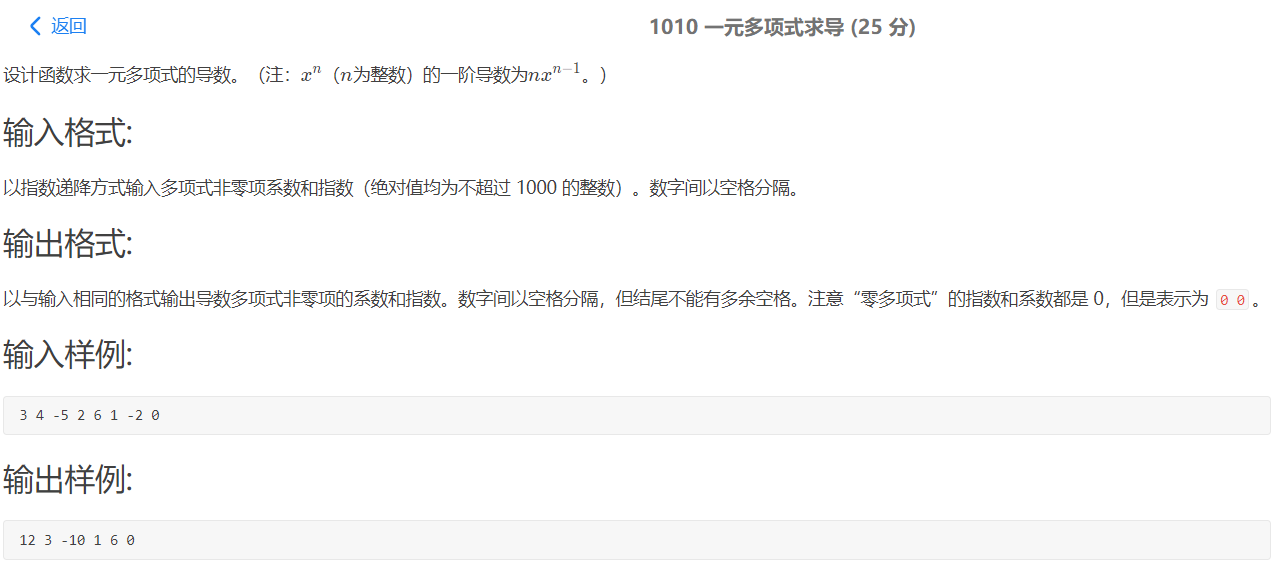• 一元多项式求导
一元多项式求导

题目描述：

设计函数求一元多项式的导数。

输入格式:

以指数递降方式输入多项式非零项系数和指数（绝对值均为不超过1000的整数）。数字间以空格分隔。

输出格式:

以与输入相同的格式输出导数多项式非零项的系数和指数。数字间以空格分隔，但结尾不能有多余空格。

输入样例:

3 4 -5 2 6 1 -2 0

输出样例:

12 3 -10 1 6 0

代码：

链表：

#include<stdio.h>
#include<stdlib.h>
#include<malloc.h>

typedef struct LNode
{
int coefficient;//系数
int exponential;//指数
struct LNode *next;
}LNode, *LinkList;

void List_Create(LinkList &L)
{
L = (LNode *)malloc(sizeof(LNode));
L->next = NULL;
}

void List_Init(LinkList &L)
{
LNode *p = L;
int a,b;
int i = 0;
while(scanf("%d %d",&a,&b) != EOF)
{
a = a * b;
b = b - 1;
if(a != 0)
{
LNode *q = (LNode *)malloc(sizeof(LNode));
q->coefficient = a;
q->exponential = b;
if(i == 0)
printf("%d %d",q->coefficient,q->exponential);
else
printf(" %d %d",q->coefficient,q->exponential);
q->next = p->next;
p->next = q;
p = q;
++i;
}
}
if(i == 0)
printf("0 0");
printf("\n");
}

int List_Destory(LinkList &L)
{
LNode *p = L;
while(p != NULL)
{
LNode *q = p->next;
free(p);
p = q;
}
return 0;
}

int main()
{
LinkList L1;
List_Create(L1);
List_Init(L1);
List_Destory(L1);
return 0;
}


简单：

#include<stdio.h>

int main()
{
int a,b;
int times = 0;
while(scanf("%d %d",&a,&b) != EOF)
{
a = a * b;
b = b - 1;
if(a == 0)
;
else if(times == 0)
{
printf("%d %d",a,b);
++times;
}
else
printf(" %d %d",a,b);
}
if(times == 0)
printf("0 0");
printf("\n");
return 0;
}

代码分析：

这道题目没有必要将数据经行存储，直接输出就可以。用链表进行操作时最好存储已经处理好的数据，或者在输出时进行判断。最复杂的就是存储好再进行删除，再输出。
展开全文链表
• 一元多项式求导代码：

参考柳婼大神思路

#include<iostream>
using namespace std;
int main(){
int a,b,flag=0;
while(cin>>a>>b){
if(b!=0){  //输入的是所有非零项系数，肯定会有输出
if(flag==1)  printf(" ");  //flag⽤用来判断是否已经有过输出
flag=1;
cout<<a*b<<" "<<b-1;
}
}
if(flag==0)
printf("0 0");
}

注意：

注意空格的输出以及只输入常数项的情况。

展开全文• 设计函数求一元多项式的导数。（注：*xn（n为整数）的一阶导数为nx**n*−1。） 输入格式: 以指数递降方式输入...// 1010 一元多项式求导.cpp : 此文件包含 main 函数。程序执行将在此处开始并结束。 // #include u
• 2-7 一元多项式求导 (20分) 设计函数求一元多项式的导数。
2-7 一元多项式求导 (20分)
设计函数求一元多项式的导数。
输入格式:
以指数递降方式输入多项式非零项系数和指数（绝对值均为不超过1000的整数）。数字间以空格分隔。
输出格式:
以与输入相同的格式输出导数多项式非零项的系数和指数。数字间以空格分隔，但结尾不能有多余空格。
输入样例:
3 4 -5 2 6 1 -2 0

输出样例:
12 3 -10 1 6 0

刚开始的时候，本能的第一反应就是用链表来写。
可是在执行的过程中发现了好多困难，结果就是下面那样子了。【哈哈，后来我改对了，但是时隔好久了】
之后吧，还是向实力低头了，用数组来写吧。最下面有正确答案。

首先啊，我用C语言来写的时候，不知道怎么来判断输入什么时候可以截止。
第二，多项式求导的时候，如果出现了一个只有常数项的东西，该项求导之后系数和指数全部为0，到底是输出0 0，还是什么也不输出啊？
事实证明，输出0 0
不急，后来借鉴之后，发现C++的代码好多人在用，不使用链表很简单，代码简洁，道理自己懂就行。

C++代码：
总结了好多答案，可以使用了。
我这真难啊，鼓捣了好几天才写完。

注意：当输入只有常数项，比如5 0 的时候，求导之后就什么都没有了，我闷习惯的认为就是0，但是这里要输出0 0。
记住吧，孩子！

#include <iostream>

using namespace std;

int main()
{
int x,y;
int flag=0;
while(scanf("%d %d",&x,&y)==2&&y!=0)
{
if(flag++)
cout<<" ";
cout<<x*y<<" "<<y-1;
}
if(!flag)
cout<<"0 0";

return 0;
}


链表【已更正】

这个是我在后来复习的时候改正的，但是这个题目集已经结束了，我把一些极端的数据都测试了一下，没什么问题了，但是我又不是PTA，如果有人碰到一样的题目，可以帮我把这个答案测试一下吗？顺便告诉我结果哦。
谢谢喽！

#include <stdio.h>
#include <stdlib.h>

typedef struct SNode {
int m;
int n;
struct SNode *Next;

} Node,*List;

List CreatList();
void PrintList(List L);
List operate(List L);

int main() {
List L;
L=CreatList();
L=operate(L);
PrintList(L);

return 0;
}
List CreatList() {
List L;
Node *p,*s;
int x,y;
L=(List)malloc(sizeof(Node));//链表的头结点
L->Next=NULL;
s=L;
while(scanf("%d %d",&x,&y)==2&&y!=0) {
p=(List)malloc(sizeof(Node));
p->m=x;
p->n=y;
s->Next=p;
s=p;

}

s->Next=NULL;
return L;

}
void PrintList(List L) {
Node *p;
p=L->Next;
if(p==NULL)
printf("0 0");

/*
我在这里说一下吧，开始的时候自己不会，末尾不能有空格，我就只会判断
什么时候是最后一个元素，我个别特殊的输出一下吧，其他的都正常。
但是后来发现了大家都在使用这种方式，我感觉还不错呢，以后就这样写了。
PS： 56-62和 64-71效果一样的啊，留一个就行了，别太贪心，会错的。
*/
int cnt=0;
while(p) {
if(cnt++)
printf(" ");
printf("%d %d",p->m,p->n);
p=p->Next;
}

/*	while(p) {
if(p->Next==NULL)
printf("%d %d",p->m,p->n);
else
printf("%d %d ",p->m,p->n);
p=p->Next;
}
*/
}
List operate(List L) {
Node *p;
p=L->Next;
while(p) {
if(p->n==0) {
p->m=0;
} else {
p->m=p->m*p->n;
p->n--;

}
p=p->Next;
}
return L;
}

数组【已更正】：
#include <stdio.h>
#include <stdlib.h>

int main() {
int x,y;
int cnt=0;

while(scanf("%d %d",&x,&y)==2) {
if(y==0&&cnt==0)
printf("0 0");
if(y==0)
break;
if(cnt++)
printf(" ");
printf("%d %d",x*y,y-1);

}
return 0;
}



展开全文链表 数据结构
• 一元多项式求导 /************************************************** 一元多项式求导 设计函数求一元多项式的导数。（注：xn（n为整数）的一阶导数为n*xn-1。） 输入描述： 以指数递降方式输入多项式非零...
一元多项式求导

/**************************************************
一元多项式求导
设计函数求一元多项式的导数。（注：xn（n为整数）的一阶导数为n*xn-1。）
输入描述：
以指数递降方式输入多项式非零项系数和指数（绝对值均为不超过1000的整数）。
数字间以空格分隔。
输出描述：
以与输入相同的格式输出导数多项式非零项的系数和指数。数字间以空格分隔，
但结尾不能有多余空格。注意“零多项式”的指数和系数都是0，但是表示为“0 0”。

**************************************************/
#include<iostream>
using namespace std;
int main()
{
int arr1;
int arr2;
int i = 0;
for (;cin >> arr1[i] >> arr2[i];++i)
{
if (arr2[i] != 0)
{
arr1[i] = arr1[i] * arr2[i]--;
}
else
{
i--;
}
}
if (i==0)//上方算法只有一个常数项时没有输出 但是需要输出0 0 所有进行一次判定
{
cout << 0 << " "<<0 << endl;
return 0;
}
for (int j = 0;j < i;++j)
{
cout << arr1[j] << " " << arr2[j];
if (j < i - 1)
{
cout << " ";
}

}
cout << endl;
return 0;
}




展开全文PAT
• 1010 一元多项式求导 https://www.cnblogs.com/xx123/p/10326143.html #include<iostream> using namespace std; int main() { int expo;//系数 int index;//指数 cin>>expo>>index; if......